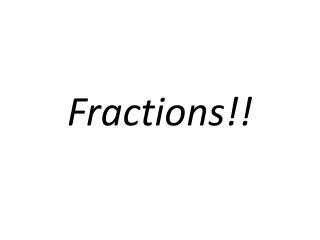DownloadDownload PresentationFractions!!

# Fractions!!

Download Presentation## Fractions!!

- - - - - - - - - - - - - - - - - - - - - - - - - - - E N D - - - - - - - - - - - - - - - - - - - - - - - - - - -
##### Presentation Transcript

1. Fractions!!

2. Prime NumbersandFactorization

3. How many positive prime numbers are less than 100?

5. What is the sum of the prime numbers between π and 10π?

7. List the positive prime numbers less than 100 that have the units digit equal to 3.

8. Answer:3, 13, 23, 43, 53, 73, 83

9. Prime Factorization

10. Factoring is like taking a number apart. It means to express a number as the product of its factors. Factors are either composite numbers or prime numbers (except that 0 and 1 are neither prime nor composite).The number 12 is a multiple of 3, because it can be divided evenly by 3.3 · 4 = 123 and 4 are both factors of 123 · 2 · 2 (prime factorization of 12)12 is a multiple of both 3 and 4.

12. Answer:2∙2∙3∙3∙3∙3∙3 or2² ∙ 3⁵(Prime-Power Factorization)

13. List the prime factorization of the following terms (includes variables): 25n²

15. List the prime factorization of the following terms (includes variables): 92xy

17. Factors

18. Answer:1, 2, 3, 5, 6, 10, 15, 30

20. Greatest Common Factor (GCF)

21. Greatest Common FactorThe highest number that divides exactly into two or more numbers. If you find all the factors of two or more numbers, and some factors are the same ("common"), then the largest of those common factors is the Greatest Common Factor.Abbreviated "GCF". Also called "Highest Common Factor"Example: the GCF of 12 and 30 is 6, because 1, 2, 3 and 6 are factors of both 12 and 30, and 6 is the greatest.

22. Find the GCF of 24 & 28

24. Find the GCF of 39v & 30uv

26. Find the GCF of 35n²m & 21m²n

28. Find the GCF of 36xy³ & 24y²

30. Find the GCF of 105x, 30yx & 75x

32. Least Common Multiple (LCM)

33. Least Common MultipleThe smallest (non-zero) number that is a multiple of two or more numbers.Least Common Multiple is made up of the words Least, Common and Multiple:What is a "Multiple" ?The multiples of a number are what you get when you multiply it by other numbers (such as if you multiply it by 1,2,3,4,5, etc). Just like the multiplication table. Here are some examples: The multiples of 3 are: 3, 6, 9, 12, 15, 18, 21, etc ... The multiples of 12 are: 12, 24, 36, 48, 60, 72, etc... What is a "Common Multiple" ?When you list the multiples of two (or more) numbers, and find the same value in both lists, then that is a common multiple of those numbers. For example, when you write down the multiples of 4 and 5, the common multiples are those that are found in both lists:The multiples of 4 are: 4,8,12,16,20,24,28,32,36,40,44,... The multiples of 5 are: 5,10,15,20,25,30,35,40,45,50,...Notice that 20 and 40 appear in both lists? So, the common multiples of 4 and 5 are: 20, 40, (and 60, 80, etc ..., too) What is the "Least Common Multiple" ?It is simply the smallest of the common multiples. In our previous example, the smallest of the common multiples is 20 ...... so the Least Common Multiple of 4 and 5 is 20.

34. Finding the Least Common MultipleIt is a really easy thing to do. Just start listing the multiples of the numbers until you get a match.Example: Find the least common multiple for 3 and 5:The multiples of 3 are 3, 6, 9, 12, 15, ..., and the multiples of 5 are 5, 10, 15, 20, ..., like this:As you can see on this number line, the first time the multiples match up is 15. Answer: 15More than 2 NumbersYou can also find the least common multiple of 3 (or more) numbers.Example: Find the least common multiple for 4, 6, and 8Multiples of 4 are: 4, 8, 12, 16, 20, 24, 28, 32, 36, ...Multiples of 6 are: 6, 12, 18, 24, 30, 36, ...Multiples of 8 are: 8, 16, 24, 32, 40, ....So, 24 is the least common multiple (I can't find a smaller one !)

35. Find the LCM of 35 & 25

37. Find the LCM of 30, 25 & 10

39. Find the LCM of 18 & 6v